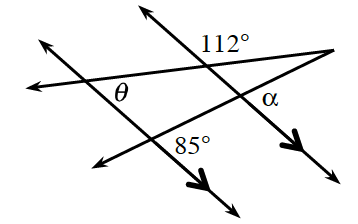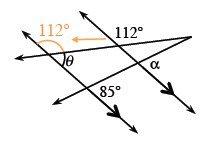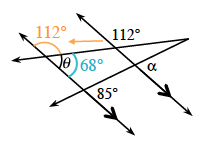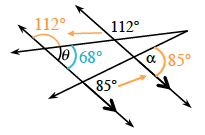### Home > CCG > Chapter 4 > Lesson 4.2.5 > Problem4-115

4-115.Find the values of $θ$ and $α$ in the diagram at right. State the relationships you used.

There are several correct methods.

The value of $θ$ can be found by using corresponding and supplementary angles.

Label added at the intersection of the left parallel line & the top transversal, on the exterior right angle, 112 degrees, with arrow pointing from corresponding 112 degree angle.

Label added to angle labeled theta, 68 degrees.

The value of $α$ can be found using corresponding angles.

Label added to angle labeled alpha, 85 degrees, with arrow pointing from corresponding 85 degree angle.